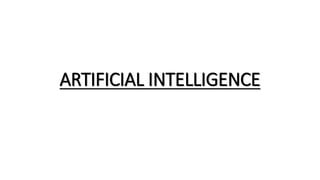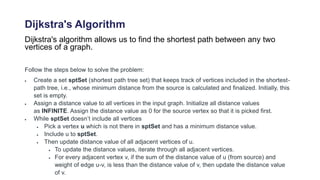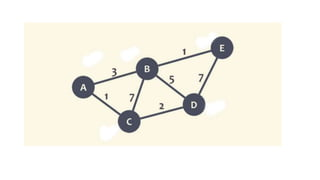Successfully reported this slideshow.

# ARTIFICIAL INTELLIGENCE.pptx×

# ARTIFICIAL INTELLIGENCE.pptx

Presentation on Artificial Intelligence and its categories. Dijkstra's Algorithm

Presentation on Artificial Intelligence and its categories. Dijkstra's Algorithm

### ARTIFICIAL INTELLIGENCE.pptx

1. 1. ARTIFICIAL INTELLIGENCE
2. 2. Dijkstra's Algorithm Dijkstra's algorithm allows us to find the shortest path between any two vertices of a graph. Follow the steps below to solve the problem:  Create a set sptSet (shortest path tree set) that keeps track of vertices included in the shortest- path tree, i.e., whose minimum distance from the source is calculated and finalized. Initially, this set is empty.  Assign a distance value to all vertices in the input graph. Initialize all distance values as INFINITE. Assign the distance value as 0 for the source vertex so that it is picked first.  While sptSet doesn’t include all vertices  Pick a vertex u which is not there in sptSet and has a minimum distance value.  Include u to sptSet.  Then update distance value of all adjacent vertices of u.  To update the distance values, iterate through all adjacent vertices.  For every adjacent vertex v, if the sum of the distance value of u (from source) and weight of edge u-v, is less than the distance value of v, then update the distance value of v.
3. 3. What is A* Search Algorithm? A* Search algorithm is one of the best and popular technique used in path-finding and graph traversals. OR A computer method used to find the optimal path between two mapped locations. A* algorithm has 3 parameters:  g : the cost of moving from the initial cell to the current cell. Basically, it is the sum of all the cells that have been visited since leaving the first cell.  h : also known as the heuristic value, it is the estimated cost of moving from the current cell to the final cell. The actual cost cannot be calculated until the final cell is reached. Hence, h is the estimated cost. We must make sure that there is never an over estimation of the cost.  f : it is the sum of g and h. So, f = g + h
4. 4. Artificial Intelligence Artificial intelligence is the simulation of human intelligence processes by machines, especially computer systems. Specific applications of AI include expert systems, natural language processing, speech recognition and machine vision.
5. 5. What are the advantages and disadvantages of artificial intelligence? Advantages Good at detail-oriented jobs; Reduced time for data-heavy tasks; Delivers consistent results; and AI-powered virtual agents are always available. Disadvantages Expensive Requires deep technical expertise; Limited supply of qualified workers to build AI tools; Only knows what it's been shown; and Lack of ability to generalize from one task to another.
6. 6. CATEGORIES OF AI Narrow AI -is when a machine has superior performance to a human when doing one specific task General AI – is when a machine is similar in its performance to a human in any intellectual task Strong AI – is when a machine has superior performance to a human in many tasks
7. 7. What is machine learning? Machine learning refers to the study of computer systems that learn and adapt automatically from experience, without being explicitly programmed. OR A computer program that improves its performance at certain task with experience. With simple AI, a programmer can tell a machine how to respond to various sets of instructions by hand-coding each “decision.” With machine learning models, computer scientists can “train” a machine by feeding it large amounts of data. The machine follows a set of rules—called an algorithm—to analyze and draw inferences from the data. The more data the machine parses, the better it can become at performing a task or making a decision. Here’s one example you may be familiar with: Music streaming service Spotify learns your music preferences to offer you new suggestions. Each time you indicate that you like a song by listening through to the end or adding it to your library, the service updates its algorithms to feed you more accurate recommendations. Netflix and Amazon use similar machine learning algorithms to offer personalized recommendations.
8. 8. What is Deep learning???? It is a machine learning technique that teaches computers to do what comes naturally to humans. OR In deep learning, a computer model learns to perform classification tasks directly from images, text, or sound.
9. 9. TYPES OF MACHINE LEARNING  Supervised Learning  Unsupervised Learning  Reinforcement Learning
10. 10. In Supervised learning, the AI model is trained based on the given input and its expected output, i.e., the label of the input. The model creates a mapping equation based on the inputs and outputs and predicts the label of the inputs in the future based on that mapping equation. Let’s suppose we have to develop a model that differentiates between a cat and a dog. To train the model, we feed multiple images of cats and dogs into the model with a label indicating whether the image is of a cat or a dog. The model tries to develop an equation between the input images and their labels. After training, the model can predict whether an image is of a cat or a dog even if the image is previously unseen by the model.
11. 11. In Unsupervised learning, the AI model is trained only on the inputs, without their labels. The model classifies the input data into classes that have similar features. The label of the input is then predicted in the future based on the similarity of its features with one of the classes. Suppose we have a collection of red and blue balls and we have to classify them into two classes. Let’s say all other features of the balls are the same except for their color. The model tries to find the dissimilar features between the balls on the basis of how the model can classify the balls into two classes. After the balls are classified into two classes depending on their color, we get two clusters of balls, one of blue color and one of red color.
12. 12. In Reinforcement learning, the AI model tries to take the best possible action in a given situation to maximize the total profit. The model learns by getting feedback on its past outcomes. Consider the example of a robot that is asked to choose a path between A and B. In the beginning, the robot chooses either of the paths as it has no past experience. The robot is given feedback on the path it chooses and learns from this feedback. The next time the robot gets into a similar situation, it can use feedback to solve the problem. For example, if the robot chooses path B and gets a reward, i.e., positive feedback, this time the robot knows that it has to choose path B to maximize its reward.
13. 13. NOTE:  ML - A computer program that improves its performance at certain task with experience.  DL – it structures algorithm in layers to create an artificial neural network.  Neural networks - try to emulate the human brain, combining computer science.  Graph theory - is the study of graphs, which are mathematical structures used to model pairwise relations between objects.  Trees - abstract data type with a hierarchical structure.